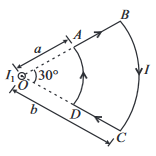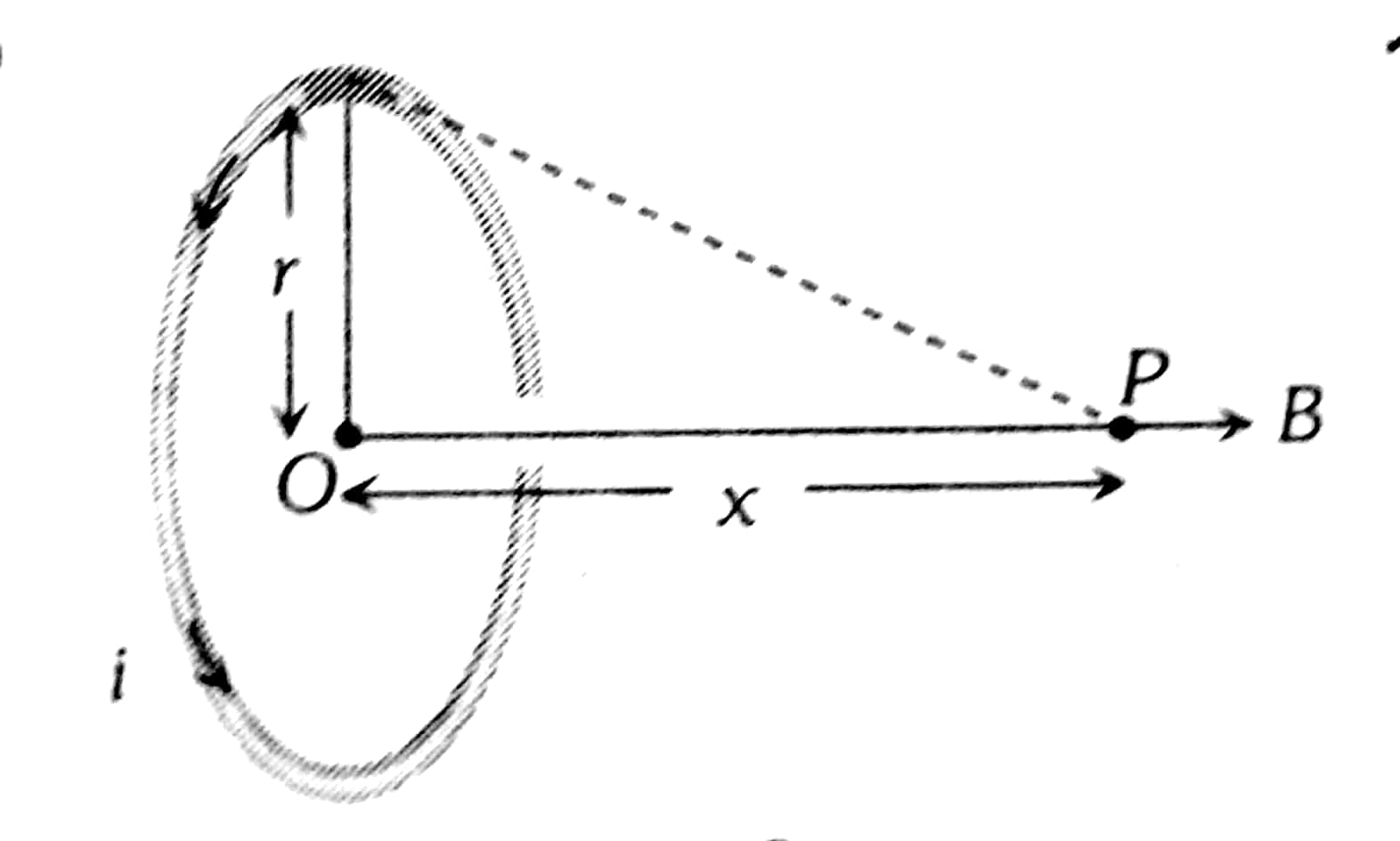Q

# Confused! kindly explain, - Magnetic Effects of Current and Magnetism - JEE Main

Directions : Question are based on the following paragraph.

A current loop is held fixed on the plane of the paper as shown in the figure. The arcs and of the loop are joined by two straight wires and . A steady current is flowing in the loop. Angle made by and at the origin is . Another straight thin wire with steady current   flowing out of the plane of the paper is kept at the origin.Question : Due to the presence of the current at the origin

• Option 1)

the forces on are zero

• Option 2)

the forces on are zero

• Option 3)

the magnitude of the net force on the loop is given by

• Option 4)

the magnitude of the net force on the loop is given by

93 Views

As we learnt in

Magnetic Field due to Circular Current at the centre -

If a coil of radius r is carrying current i then magnetic feild at a distnace X from its centre is:

- whereinThe force on AD and BC due to current  is zero.

This is because the directions of current element  and magnetic field  are parallel.

Option 1)

the forces on are zero

Incorrect

Option 2)

the forces on are zero

Correct

Option 3)

the magnitude of the net force on the loop is given by

Incorrect

Option 4)

the magnitude of the net force on the loop is given by

Incorrect

Exams
Articles
Questions